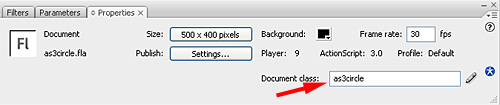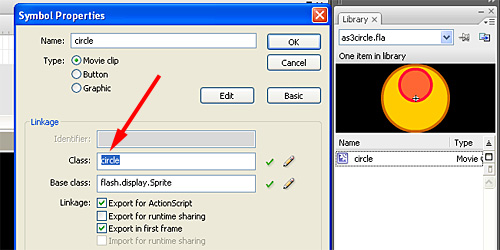# Flash game creation tutorial – part 1 (with AS3 classes)

Old readers should remember Flash game creation tutorial – part 1… it’s an ond AS2 tutorial about the creation of a game like Ball Revamped and its sequels.

Later Tim Edelaar coded it into AS3 in Step by step AS3 translation of Flash game creation tutorial – part 1, but with the whole code in a single class, and now it’s time for me to release the AS3 version of the tutorial using classes.

We are going to use three actionscript files: `as3circle.as` for the game, `circle.as` for the hero (the ball) and `keys.as` for keyboard input.

`keys.as` is the same class used in Introduction to AS3 classes so you can understand the importance of writing classes: I won’t have to worry for keyboard input anymore (well… as long as I am using arrows and space keys)

Linking classes is very important in AS3, that’s how you should manage class linking:

This is the movie properties panel:Writing `as3circle` in the Document class field will set `as3circle.as` as the main class file.

This is the circle object properties panel:Writing `circle` in the Class field will set circle.as as the class file for the circle object.

Now your `.fla` file and the three `.as` files must be in the same path.

And this is the code for every file… I don’t think there is any need to comment it because it was already commented in dozens of posts… anyway if you need comments, just ask for them in… the comments.

`as3circle.as` file:

```package {
import flash.display.Sprite;
import flash.events.Event;
public class as3circle extends Sprite {
public var keyboard_input:keys;
public var circle_hero = new circle;
public function as3circle() {
circle_hero.init();
var keyboard_sprite = new Sprite();
keyboard_input = new keys(keyboard_sprite);
}
public function on_enter_frame(event:Event) {
if (keyboard_input.is_left()) {
circle_hero.apply_force(-1,0);
}
if (keyboard_input.is_right()) {
circle_hero.apply_force(1,0);
}
if (keyboard_input.is_up()) {
circle_hero.apply_force(0,-1);
}
if (keyboard_input.is_down()) {
circle_hero.apply_force(0,1);
}
}
}
}```

`circle.as` file:

```package {
import flash.display.Sprite;
import flash.events.Event;
public class circle extends Sprite {
private var x_speed:Number;
private var y_speed:Number;
private var power:Number;
private var friction:Number;
private var gravity:Number;
public function circle() {
}
private function movement(e:Event) {
x+=x_speed;
y+=y_speed;
rotation += x_speed;
y_speed += gravity;
x_speed *= friction;
y_speed *= friction;
if (x>500 || x<0 ||  y >400 || y<0) {
init();
}
}
public function apply_force(x_force,y_force) {
x_speed += (x_force*power);
y_speed += (y_force*power);
}
public function init() {
gravity = 0.1;
power = 0.66;
friction = 0.99;
x_speed = 0;
y_speed = 0;
x = 250;
y = 200;
}
}
}```

`keys.as` file

```package {
import flash.events.KeyboardEvent;
public class keys {
private var press_left = false;
private var press_right = false;
private var press_up = false;
private var press_down = false;
private var press_space = false;
public function keys(movieclip) {
}
public function is_left() {
return press_left;
}
public function is_right() {
return press_right;
}
public function is_up() {
return press_up;
}
public function is_down() {
return press_down;
}
public function is_space() {
return press_space;
}
private function key_down(event:KeyboardEvent) {
if (event.keyCode == 32) {
press_space = true;
}
if (event.keyCode == 37) {
press_left = true;
}
if (event.keyCode == 38) {
press_up = true;
}
if (event.keyCode == 39) {
press_right = true;
}
if (event.keyCode == 40) {
press_down = true;
}
}
private function key_up(event:KeyboardEvent) {
if (event.keyCode == 32) {
press_space = false;
}
if (event.keyCode == 37) {
press_left = false;
}
if (event.keyCode == 38) {
press_up = false;
}
if (event.keyCode == 39) {
press_right = false;
}
if (event.keyCode == 40) {
press_down = false;
}
}
}
}```

And we have our oooold game running in AS3 with classes.

214 GAME PROTOTYPES EXPLAINED WITH SOURCE CODE
// 1+2=3
// 10000000
// 2 Cars
// 2048
// Avoider
// Ballz
// Block it
// Blockage
// Bloons
// Boids
// Bombuzal
// Breakout
// Bricks
// Columns
// CubesOut
// Dots
// DROP'd
// Dudeski
// Eskiv
// Filler
// Fling
// Globe
// HookPod
// Hundreds
// InkTd
// Iromeku
// Lumines
// Magick
// MagOrMin
// Maze
// Memdot
// Nano War
// Nodes
// o:anquan
// Ononmin
// Pacco
// Phyballs
// Platform
// Poker
// Pool
// Poux
// Pudi
// qomp
// Racing
// Renju
// SameGame
// Security
// Sling
// Slingy
// Sokoban
// Splitter
// Sproing
// Stack
// Stringy
// Sudoku
// Tetris
// Threes
// Toony
// Turn
// TwinSpin
// vvvvvv
// Wordle
// Worms
// Yanga
// Zhed
// zNumbers